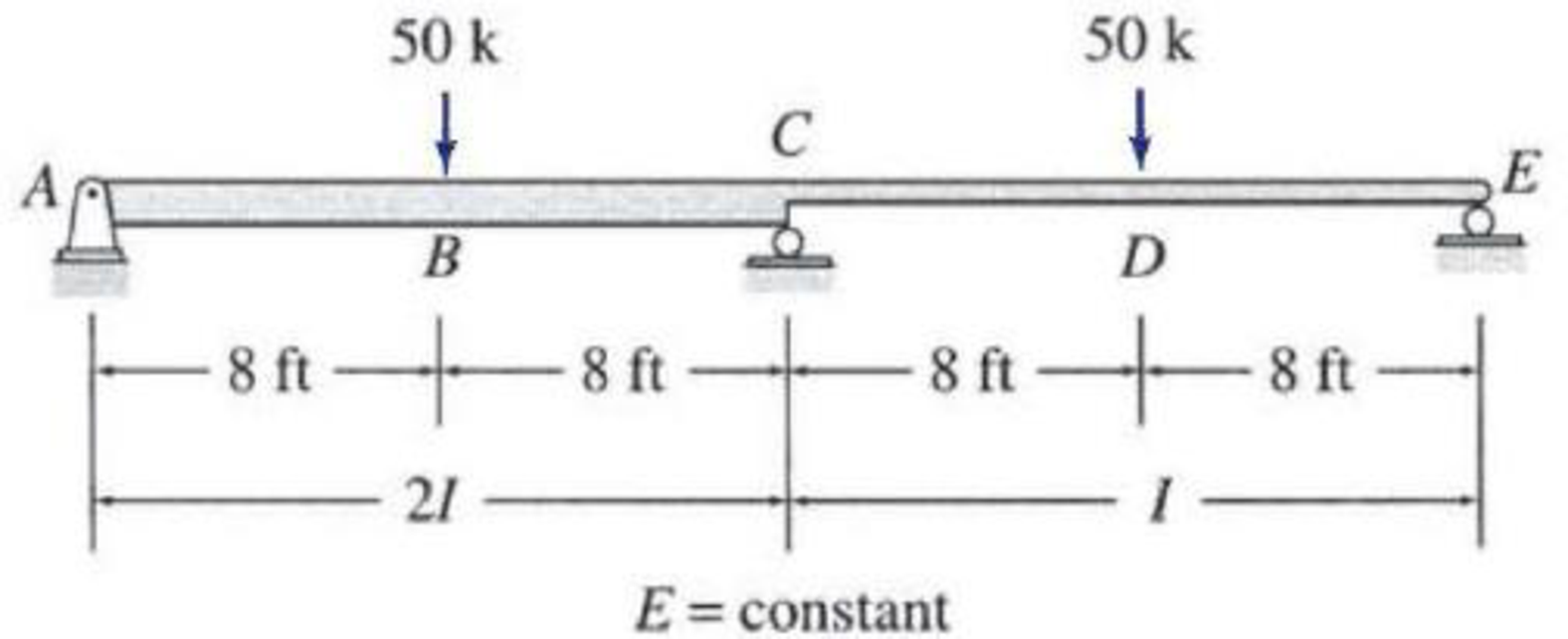# 13.30 through 13.33 Solve Problems 13.9 through 13.12 by selecting the bending moment at the interior support to be the redundant. See Figs. P13.9–P13.12. 13.9 through 13.12 Determine the reactions and draw the shear and bending moment diagrams for the beams shown in Figs. P13.9–P13.12 using the method of consistent deformations. Select the reaction at the interior support to be the redundant. FIG. P13.11, P13.32

#### Solutions

Chapter
Section
Chapter 13, Problem 32P
Textbook Problem
1 views

## 13.30 through 13.33 Solve Problems 13.9 through 13.12 by selecting the bending moment at the interior support to be the redundant. See Figs. P13.9–P13.12.13.9 through 13.12 Determine the reactions and draw the shear and bending moment diagrams for the beams shown in Figs. P13.9–P13.12 using the method of consistent deformations. Select the reaction at the interior support to be the redundant.FIG. P13.11, P13.32To determine

Calculate the support reactions for the given beam using method of consistent deformation.

Sketch the shear and bending moment diagrams for the given beam.

### Explanation of Solution

Given information:

The structure is given in the Figure.

Apply the sign conventions for calculating reactions, forces and moments using the three equations of equilibrium as shown below.

• For summation of forces along x-direction is equal to zero (Fx=0), consider the forces acting towards right side as positive (+) and the forces acting towards left side as negative ().
• For summation of forces along y-direction is equal to zero (Fy=0), consider the upward force as positive (+) and the downward force as negative ().
• For summation of moment about a point is equal to zero (Matapoint=0), consider the clockwise moment as negative and the counter clockwise moment as positive.

Calculation:

Find the degree of indeterminacy of the structure:

Degree of determinacy of the beam is equal to the number of unknown reactions minus the number of equilibrium equations.

The beam is supported by 4 support reactions and the number of equilibrium equations is 3.

Therefore, the degree of indeterminacy of the beam is i=1.

Select the bending moment MC at the interior support C as redundant.

Let θCL and θCR are the slopes at the ends C of the left and right spans of the primary beam due to external loading.

Let fCCL and fCCR are the flexibility coefficient representing the slopes at C of the left and right spans due to unit value of redundant MC.

Sketch the primary beam subjected to external loading as shown in Figure 1.

Sketch the primary beam loaded with redundant MC as shown in Figure 2.

The slope for the beam carrying central point load is expressed as,

θ=WL26EI

Here, W is the point load and L is the length of the span.

For span AC,

Substitute 50 k for W, 16 ft for L, and 2I for I.

θCL=50(16)216E(2I)=400k-ft2EI

For span CE,

Substitute 50 k for W and 16 ft for L.

θCR=50(16)216EI=800k-ft2EI

The change of slope between the two tangents due to external load (θCOrel.) is expressed as,

θCOrel.=θCL+θCR

Substitute 400k-ft2EI for θCL and 800k-ft2EI for θCR.

θCOrel.=400k-ft2EI+800k-ft2EI=1,200k-ft2EI

The flexibility coefficient fCCL is expressed as,

fCCL=L3EI

Substitute 16 ft for L and 2I for I.

fCCL=16ft3E(2I)=2.67ftEI

The flexibility coefficient fCCR is expressed as,

fCCR=L3EI

Substitute 16 ft for L.

fCCR=16ft3EI=5.33ftEI

Similarly the flexibility coefficient is fCCrel.=fCCL+fCCR.

Substitute 2.67ftEI for fCCL and 5.33ftEI for fCCR.

fCCrel

### Still sussing out bartleby?

Check out a sample textbook solution.

See a sample solution

#### The Solution to Your Study Problems

Bartleby provides explanations to thousands of textbook problems written by our experts, many with advanced degrees!

Get Started

Find more solutions based on key concepts
Present Example 11.5 in Chapter 11 using the format discussed in Section 4.2. Divide the example problem into G...

Engineering Fundamentals: An Introduction to Engineering (MindTap Course List)

Convert 5 megawatts of power into BTU/hr, ft-lbs/s, and kJ/hr.

Fundamentals of Chemical Engineering Thermodynamics (MindTap Course List)

What does RAID stand for, and what are some commonly used RAID levels?

Database Systems: Design, Implementation, & Management

Only one _____ exists on a network using STP.

Network+ Guide to Networks (MindTap Course List)

Explain the issues surrounding use of criminal databases.

Enhanced Discovering Computers 2017 (Shelly Cashman Series) (MindTap Course List)

Why is it necessary to continue to maintain and improve an existing Web site?

Principles of Information Systems (MindTap Course List)

Explain the major diameter of a thread.

Precision Machining Technology (MindTap Course List)

Describe the relation between voltage and amperage for welding current.

Welding: Principles and Applications (MindTap Course List)

If your motherboard supports ECC DDR3 memory, can you substitute non-ECC DDR3 memory?

A+ Guide to Hardware (Standalone Book) (MindTap Course List)